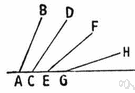# oblique angle

(redirected from Dutch angle)
Also found in: Thesaurus, Encyclopedia, Wikipedia.

## oblique angle

n.
An angle, such as an acute or obtuse angle, that is not a right angle or a multiple of a right angle.

## oblique angle

n
(Mathematics) an angle that is not a right angle or any multiple of a right angle
Collins English Dictionary – Complete and Unabridged, 12th Edition 2014 © HarperCollins Publishers 1991, 1994, 1998, 2000, 2003, 2006, 2007, 2009, 2011, 2014

## oblique′ an′gle

n.
an angle that is not a right angle; an acute or obtuse angle.
[1685–95]
 Noun 1oblique angle - an angle that is not a right angle or a multiple of a right angleangle - the space between two lines or planes that intersect; the inclination of one line to another; measured in degrees or radiansacute angle - an angle less than 90 degrees but more than 0 degreesobtuse angle - an angle between 90 and 180 degreesright angle - the 90 degree angle between two perpendicular lines